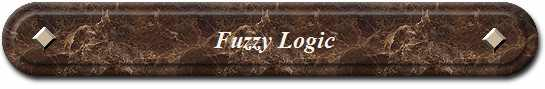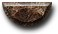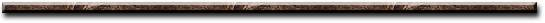News Science Technology Contact Index
 What Is It? Fuzzy Logic is based on a system of non-digital (continuous) inputs and rules. It is an approach to computing based on "degrees of truth" rather than the usual "true or false" (1 or 0) Boolean logic on which the modern computer is based. Fuzzy Logic includes 0 and 1 as extreme cases of truth (or "the state of matters" or "fact") but also includes the various states of truth in between so that, for example, the result of a comparison between two things could be not "tall" or "short" but ".38 of tallness." What Does It Do? Fuzzy Logic uses mechanical movement to represent continuous inputs that perform Boolean and mechanical operations. (Examples: cruise control, heat control, motion control, volume control) How Does It Work? Fuzzy Logic incorporates a simple, rule-based IF X AND Y THEN Z approach to solving a control problem rather than attempting to model a system mathematically. It is empirically-based, relying on an operator's experience rather than their technical understanding of the system. Example: Auto motive Speed Controller 3 inputs : speed (5 levels) acceleration (3 levels) distance to destination (3 levels) 1 output: power (fuel flow to engine) IF speed is TOO SLOW and acceleration is DECELERATING, THEN INCREASE POWER GREATLY IF speed is SLOW and acceleration is DECREASING, THEN INCREASE POWER SLIGHTLY IF distance is CLOSE, THEN DECREASE POWER SLIGHTLY ... Fuzzy Logic requires some numerical values in order to operate such as what is considered SLOW and TOO SLOW, but exact values of these numbers are usually not critical unless very responsive performance is required in which case empirical tuning would determine them. These values don't have to be symmetrical and can be "tweaked" once the system is operating in order to optimize performance. Generally, Fuzzy Logic is so forgiving that the system will probably work the first time without any tweaking.[News] [Science] [Technology] [Contact] [Index]
 Contact email: qooljaq@qooljaq.com